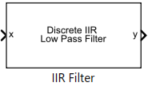# IIR Filter

Implement infinite impulse response (IIR) filter

Since R2020a

•Libraries:
Motor Control Blockset / Signal Management
Motor Control Blockset HDL Support / Signal Management

## Description

The IIR Filter block implements a discrete first-order infinite impulse response (IIR) filter on the specified input signal. The block supports fixed-point and floating-point data types. The block is also optimized for code generation when used with the model settings and configuration adopted by the examples shipped in Motor Control Blockset™.

We recommend that you use fixed-step discrete solver for this block to enable code generation and ensure accurate simulation.

### Equations

You can configure the IIR filter by using the filter coefficient ($a$) block parameter to set the required cutoff frequency (fc) for the filter.

This equation describes computation of the filter coefficient from the cutoff frequency:

Alternatively, the block also computes the theoretical cutoff frequency for the given sample time using a filter coefficient:

Use the Filter type parameter to configure the block either as a low-pass or high-pass filter.

`Low-pass filter:`

`$y\left(k\right)=a\cdot x\left(k\right)+\left(1-a\right)\cdot y\left(k-1\right)$`

`High-pass filter:`

where:

• fc is the cutoff frequency of the IIR filter.

• $a$ is the filter coefficient in the range (0, 1].

• $y\left(k\right)$ is the filtered output value at time sample $k$.

• $y\left(k-1\right)$ is the filtered output value at time sample $k-1$.

• $x\left(k\right)$ is the sampled input value at time sample $k$.

• $x\left(k-1\right)$ is the filtered output value at time sample $k-1$.

• Ts is the sample time of the IIR Filter block.

## Ports

### Input

expand all

Sampled values of the raw input signal in the time domain.

Data Types: `single` | `double` | `fixed point`

### Output

expand all

Filtered output signal returned by the IIR Filter block in the time domain.

Data Types: `single` | `double` | `fixed point`

## Parameters

expand all

Type of the IIR filter.

Filter coefficient of the IIR filter. The data type of this parameter is the same as that of the input signal. We suggest that you check the precision of the parameter value in this data type.

Select this parameter for the block to display the Discrete step size (s) and Theoretical cutoff frequency (Hz) parameters.

The fixed time interval (in seconds) between every two consecutive instances of block execution.

#### Dependencies

To display this parameter, select the Display cutoff frequency parameter.

Theoretical cutoff frequency (in Hertz) of the IIR filter. This parameter is not configurable.

#### Dependencies

To display this parameter, select the Display cutoff frequency parameter.

## Version History

Introduced in R2020a